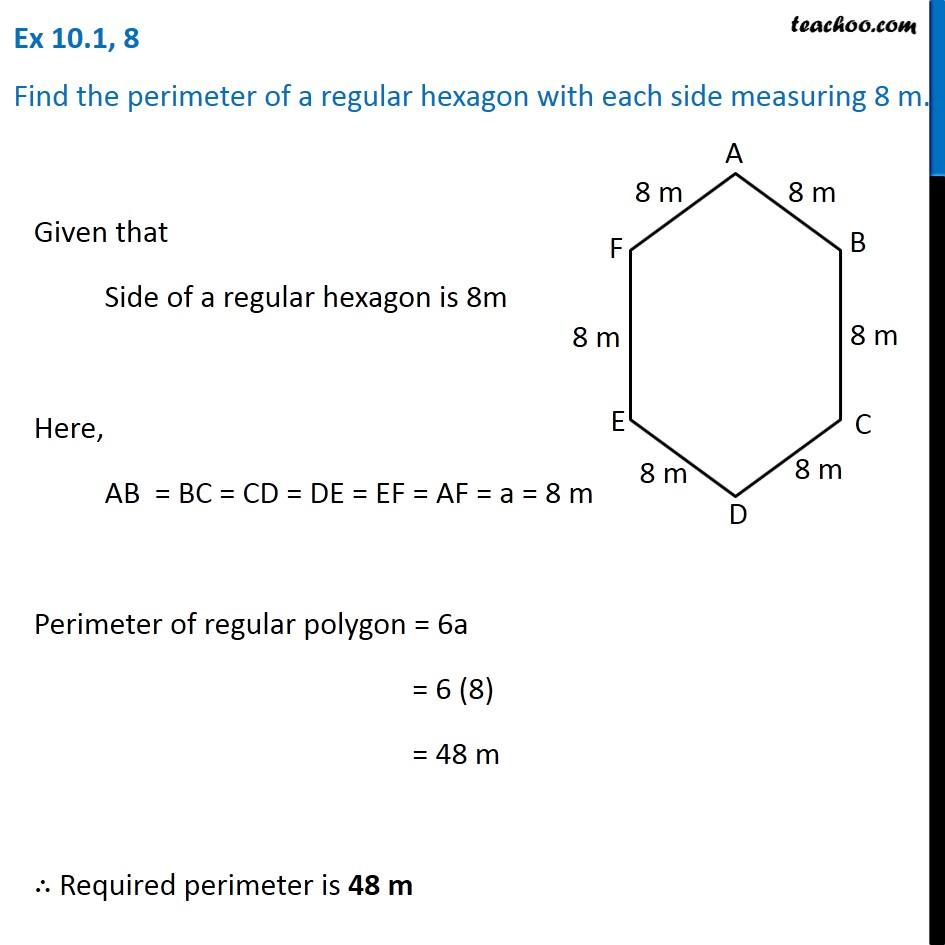Ex 10.1

Chapter 10 Class 6 Mensuration
Serial order wiseLearn in your speed, with individual attention - Teachoo Maths 1-on-1 Class

### Transcript

Ex 10.1, 8 Find the perimeter of a regular hexagon with each side measuring 8 m. Given that Side of a regular hexagon is 8m Here, AB = BC = CD = DE = EF = AF = a = 8 m Perimeter of regular polygon = 6a = 6 (8) = 48 m ∴ Required perimeter is 48 m